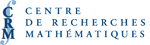# COMMANDITESUniversité Western Ontario
Département de mathématiques
Faculté d'éducation
Faculté des sciences
Recherche Western
Département de mathématiques appliquées

Nous remercions chaleureusement ces commanditaires de leur soutien.

Théorie d'Iwasawa
Org: Manfred Kolster et Romyar Sharifi (McMaster)
[PDF]

MAHESH AGARWAL, McMaster University, Dept. of Mathematics, 1280 Main St. West, Hamilton, ON L8S 4K1
[PDF]

Let p be an odd prime. In this talk we will construct a p-adic analog of a degree eight L-function L(s, F×f) where F is an ordinary holomorphic degree 2 Siegel eigen cusp form of level a power of p and f is an ordinary eigen cusp form of level a power of p.

Our method makes use of the work of M. Furusawa which gives an integral representation for this L-function. By suitably interpreting this integral representation in the context of inner products of automorphic forms, we show that it p-adically interpolates the L-values as the forms F and f vary in ordinary families (with the weights varying p-adically). This interpolation is carried out by constructing an Eisenstein measure on a higher-rank unitary group and exploiting a pull-back formula of P. Garrett and G. Shimura.

BEN HOWARD, Boston College, Chesnut Hill, MA, USA
Intersection theory on Shimura surfaces
[PDF]

Kudla, Rapoport, and Yang and have proved the equality of two modular forms of weight 3/2. One is an Eisenstein series, and the other is a formal q-expansion which encodes the arithmetic intersection numbers of CM points on a Shimura curve. Using this equality of modular forms those authors deduce a formula relating the height of a CM point in a modular Jacobian to the central derivative of an L-series, much in the spirit of the Gross-Zagier theorem. I will discuss work in progress toward a similar result for Shimura surfaces, relating the intersection multiplicities of special cycles to the Fourier coefficients of a Hilbert modular Eisenstein series of parallel weight 3/2.

A Jacquet-Langlands correspondence for p-adic families of modular
[PDF]

The simplest case of the Jacquet-Langlands correspondence associates to every cuspidal eigenform f of weight k > 2 on G0 (pl), for p,l distinct prime integers, which is new at l an eigenform g of the same weight on the quaternion algebra B of discriminant l¥, such that the Hecke eigenvalues of f and g for all Hecke operators Tn, where n is prime to l, are the same.

We propose to use vanishing cycles for p-adic cohomology on the modular curves X0 (pl) over Zl to extend this correspondence to p-adic families of modular eigenforms for G0 (pl) and B.

JENNIFER JOHNSON-LEUNG, University of Idaho, 300 Brink Hall, Moscow, ID 83844
The equivariant main conjecture of Iwasawa theory for imaginary quadratic fields
[PDF]

The main conjecture of Iwasawa theory has been a crucial tool for proving theorems about special values of L-functions. In Rubin's 1991 paper, he proved this conjecture for imaginary quadratic fields, subject to certain restrictions. To remove these restrictions, we employ a philosophy of Huber and Kings which exploits the relationship between the Tamagawa number conjecture and the main conjecture. We formulate an equivariant main conjecture for the two-variable Iwasawa algebra over an abelian extension of an imaginary quadratic field, and prove that the conjecture holds whenever the m-invariant of the class group vanishes, which it is known to do when the prime is split.

This is joint work with Guido Kings.

BYOUNG-DU KIM, Northwestern University, 2033 Sheridan Road, Evanston, Illinois, USA
Iwasawa theory for supersingular primes and the parity conjecture
[PDF]

In the study of elliptic curves and modular forms, non-ordinary primes often add extra difficulties. Thanks to Kobayashi-Perrin-Riou construction of the plus/minus universal norms, we have a reasonable Iwasawa theoretical tool to study the Selmer groups of elliptic curves for supersingular primes.

We will discuss the proof of the parity conjecture of elliptic curves for supersingular primes using this new tool, and continue to discuss possible developments for modular forms of higher weights.

KUMAR MURTY, University of Toronto, 40 St. George Street, Toronto
Growth of Selmer ranks of Abelian varieties with complex multiplication
[PDF]

We consider the growth of the Selmer rank of Abelian varieties with complex multiplication as we vary over a tower of fields. We prove two results, the first of which is in the context of an Iwasawa tower, and the second of which is for a tower of unramified extensions.

JEEHOON PARK, McGill University, Room 1126, Department of Mathematics and Statistics, Burnside Hall, 805 Sherbrooke Street West, Montreal, QC H3A 2K6
[PDF]

Some part of the results in this talk is a joint work with Glenn Stevens.

AL WEISS, University of Alberta
Congruences between pseudomeasures and Iwasawa theory
[PDF]

A refinement of the Main Conjecture of Iwasawa theory at an odd prime l has been formulated for Galois l-extensions K/k whenever k has finite degree over the rational number field and K has finite degree over its cyclotomic l-extension. Assuming that the mu-invariant of K/k is 0, it is known that this conjecture, up to its uniqueness assertion, is equivalent to two hypothetical congruences, one `logarithmic', and the other `torsion'. It had been expected that the logarithmic congruence would be more accessible than the torsion.

Recently, however, the torsion congruence has been proved in full generality. In fact, it follows quite naturally from the results of Deligne-Ribet interpreted in the pseudomeasure language of Serre.

This is joint work with J. Ritter.

BEI ZHANG, Institute for Advanced Study
Nonvanishing mod p of Eisenstein series
[PDF]

Ribet's idea about the congruence argument between automorphic forms turns out to be useful in the proof towards one direction of Iwasawa main conjecture. In the proof, a p-integral Eisenstein series E needs to be constructed so that E\not º 0 (mod p). I will recall in several cases (GSp(4) by Urban and U(2,1) by Mainardi), how this problem was solved, then explain the difficulty of obtaining such result for an Eisenstein series on U(3,1). At last, I will show the result we have so far through the calculation of Fourier-Jacobi coefficient of this Eisenstein series.